Miscellaneous

Chapter 16 Class 11 Probability
Serial order wise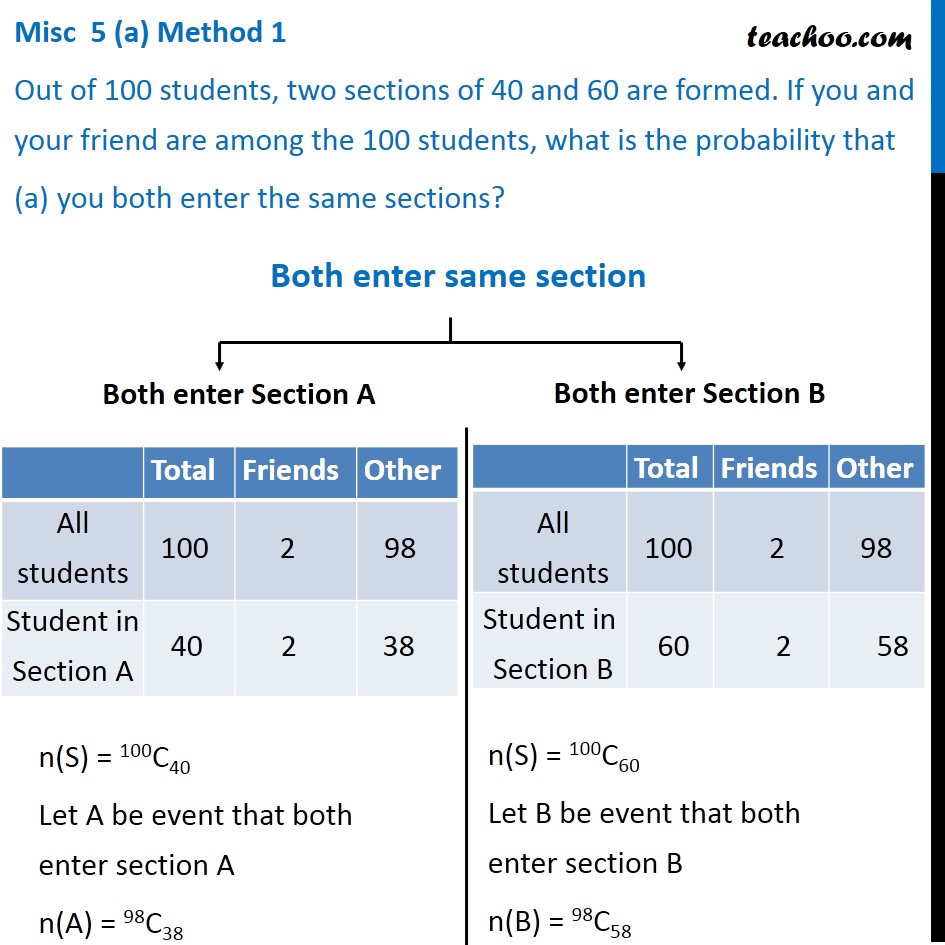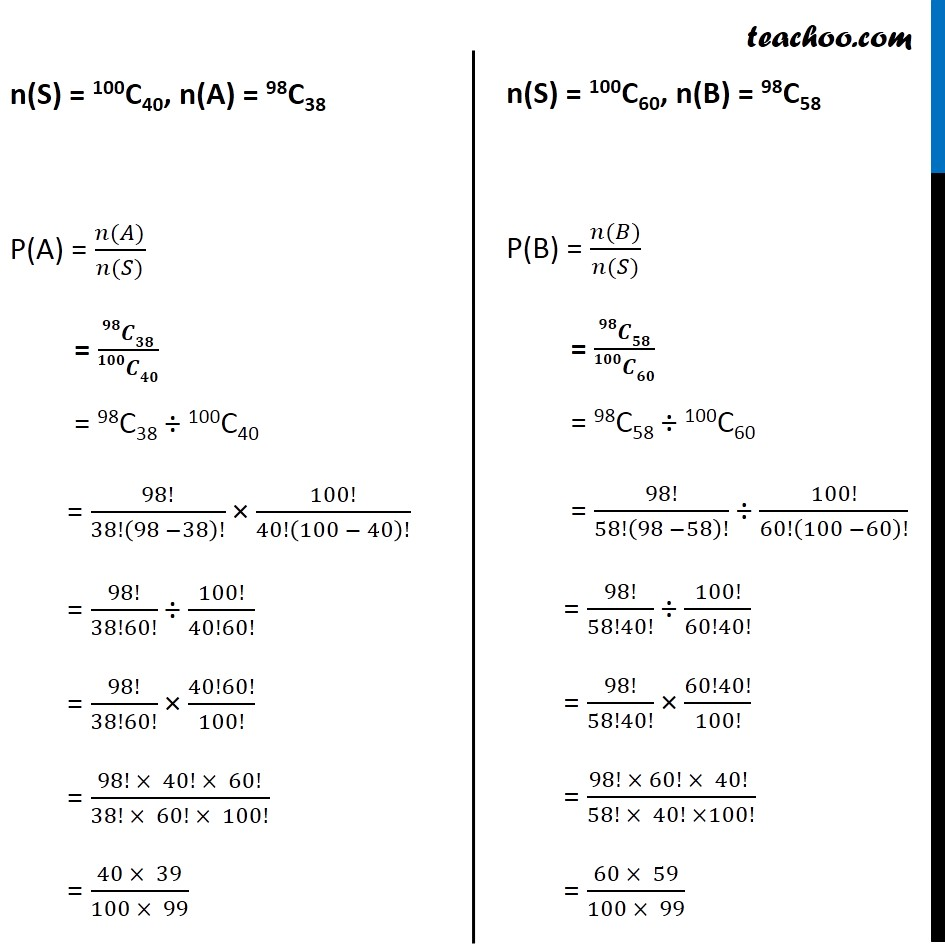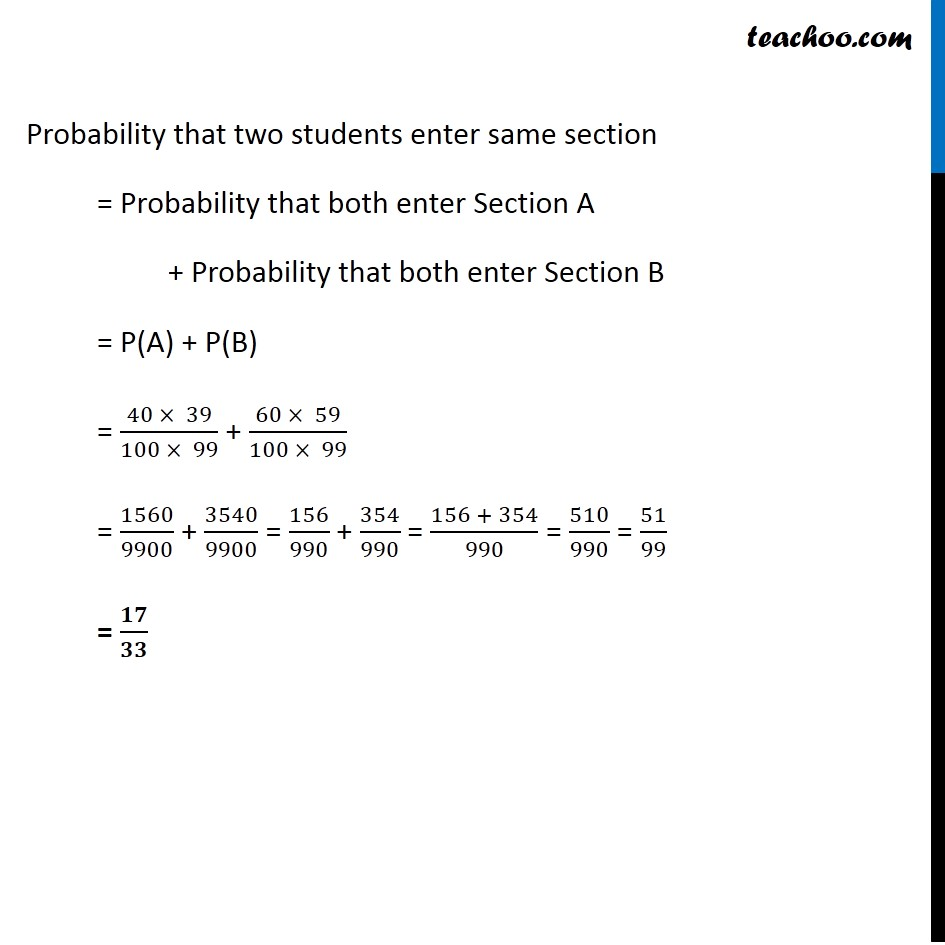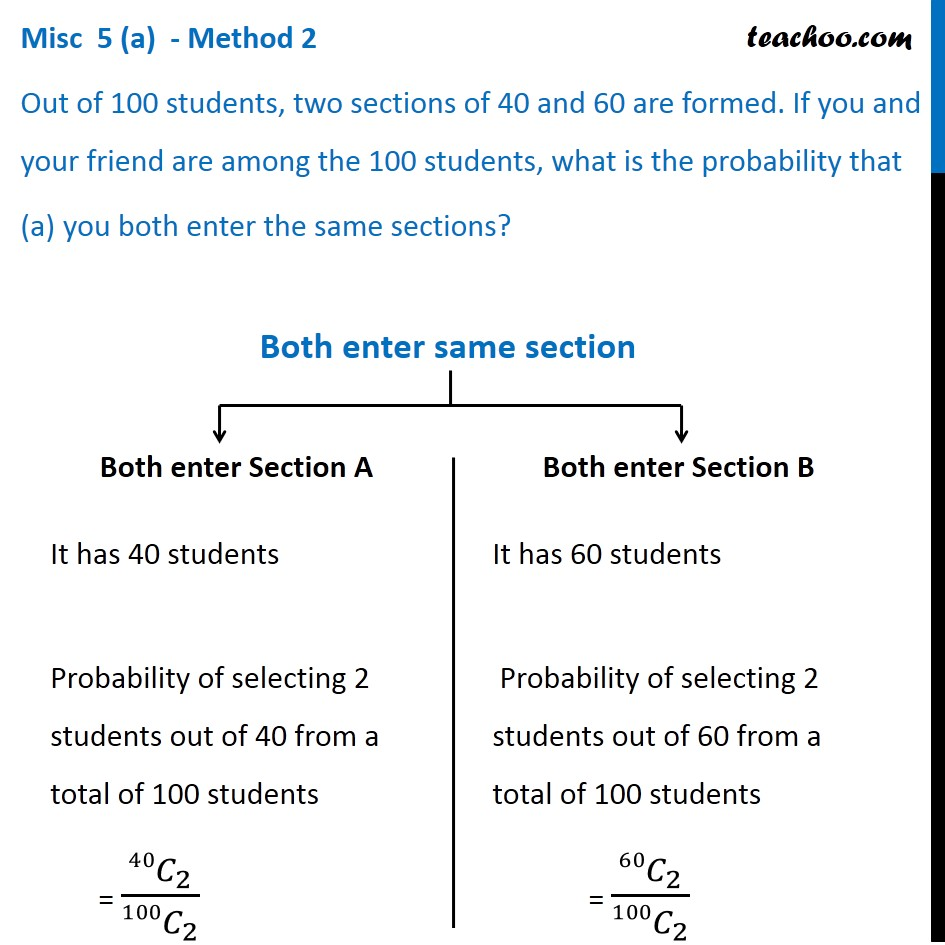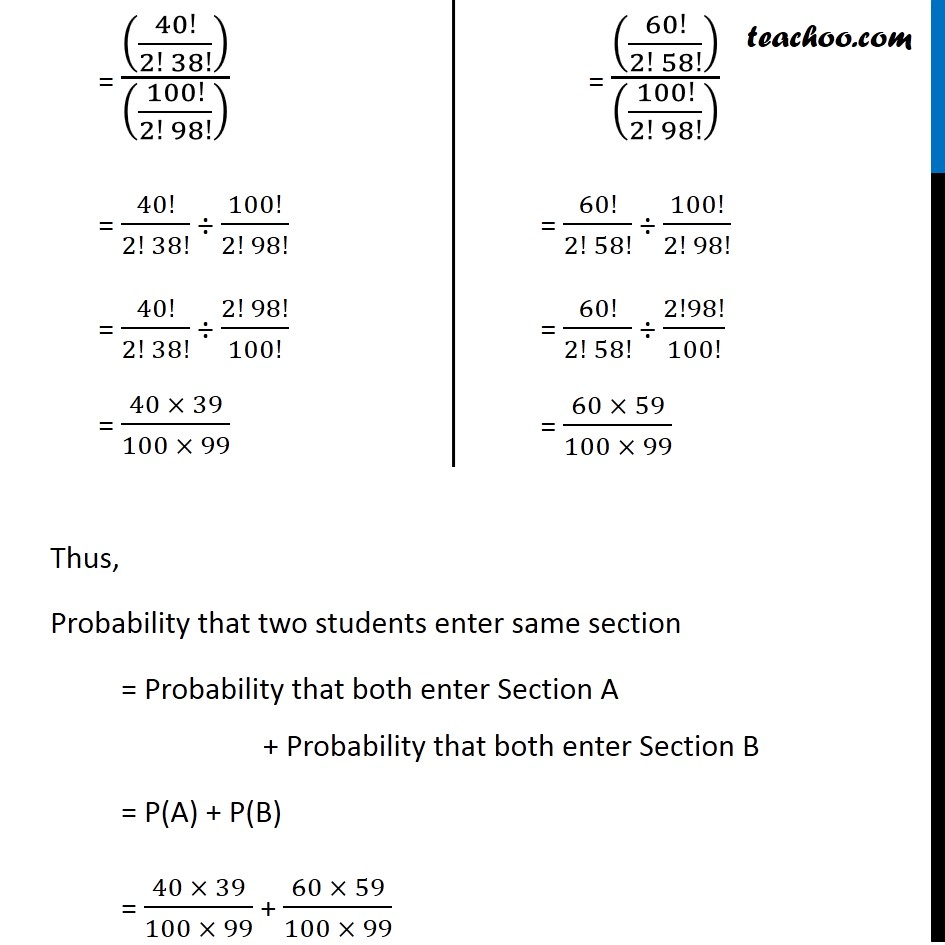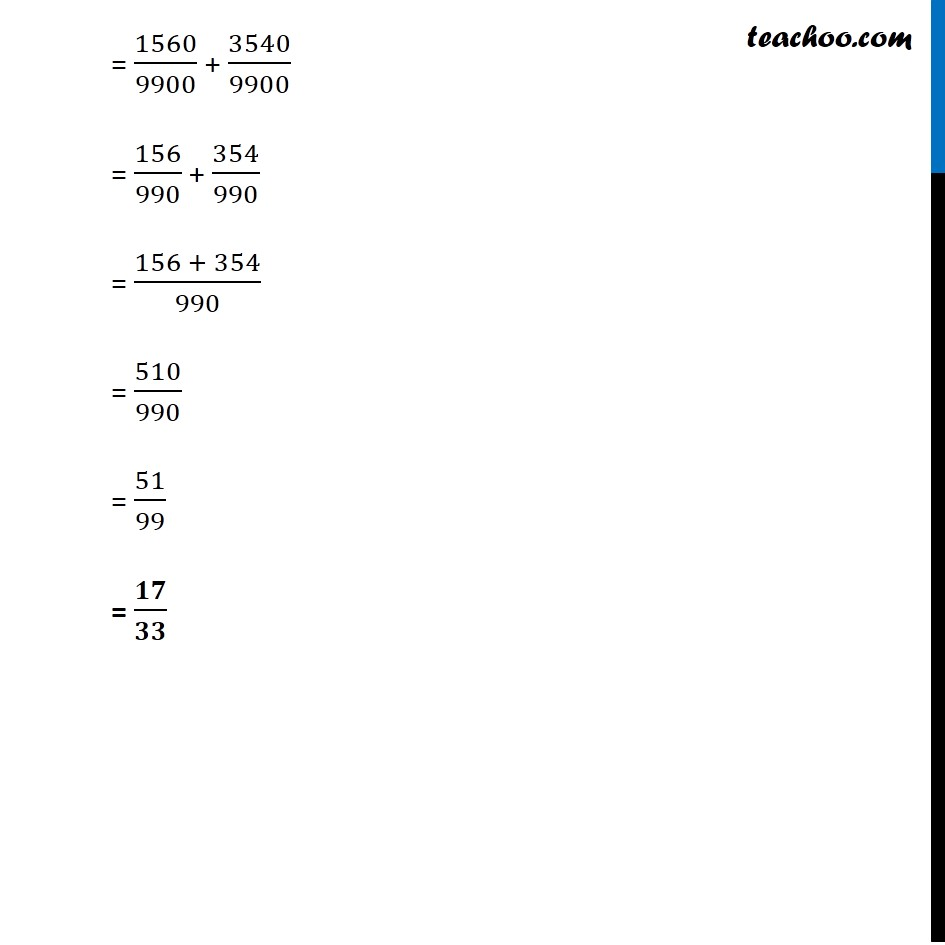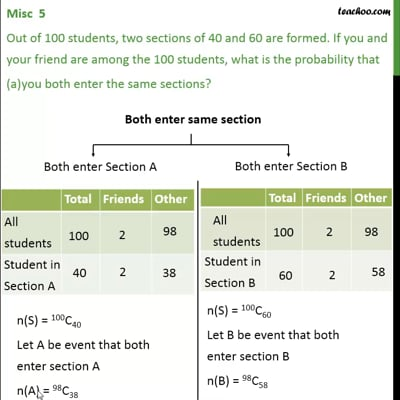This video is only available for Teachoo black users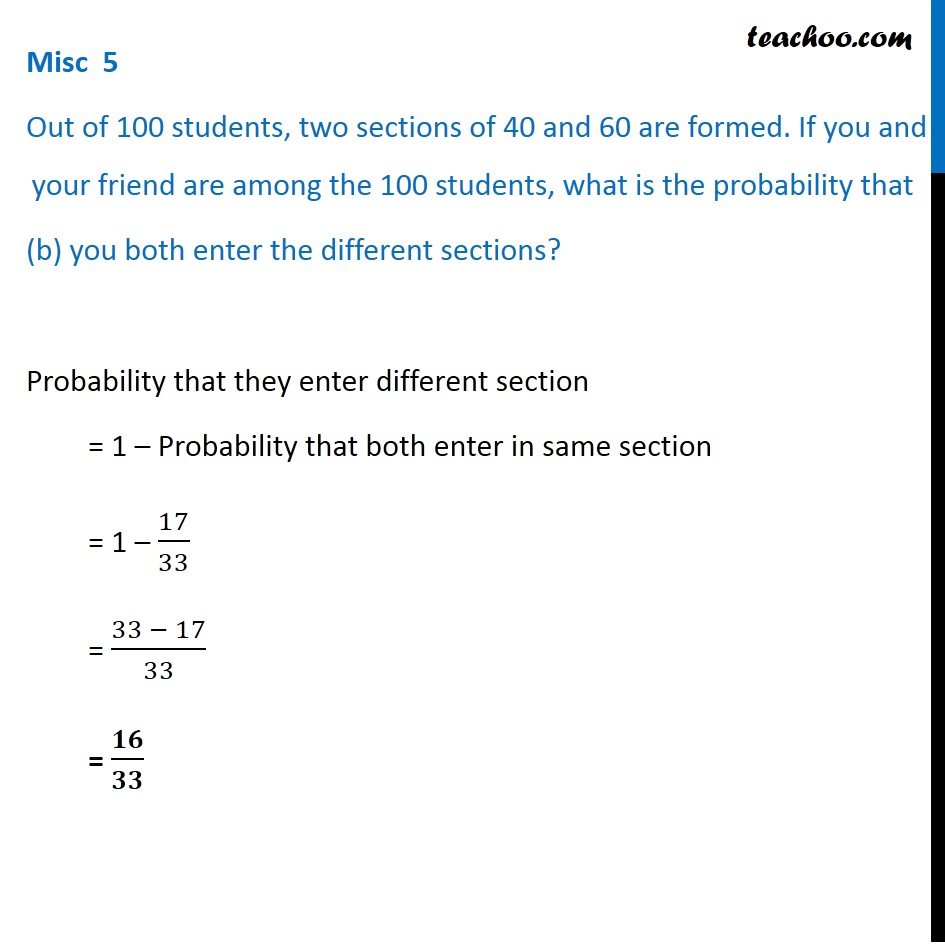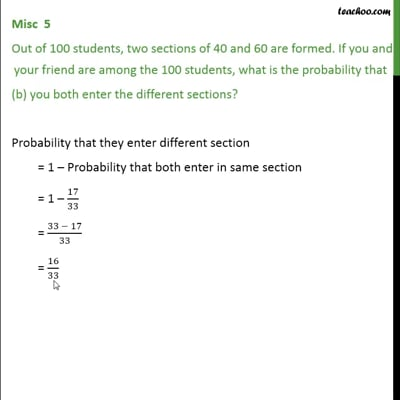This video is only available for Teachoo black users

Maths Crash Course - Live lectures + all videos + Real time Doubt solving!

### Transcript

Misc 5 (a) Method 1 Out of 100 students, two sections of 40 and 60 are formed. If you and your friend are among the 100 students, what is the probability that you both enter the same sections? n(S) = 100C40 Let A be event that both enter section A n(A) = 98C38 n(S) = 100C60 Let B be event that both enter section B n(B) = 98C58 n(S) = 100C40, n(A) = 98C38 P(A) = (𝑛(𝐴))/(𝑛(𝑆)) = 𝟗𝟖𝑪𝟑𝟖/𝟏𝟎𝟎𝑪𝟒𝟎 = 98C38 ÷ 100C40 = 98!/38!(98 −38)! × 100!/40!(100 − 40)! = 98!/38!60! ÷ 100!/40!60! = 98!/38!60! × 40!60!/100! = (98! × 40! × 60!)/(38! × 60! × 100!) = (40 × 39)/(100 × 99) n(S) = 100C60, n(B) = 98C58 P(B) = (𝑛(𝐵))/(𝑛(𝑆)) = 𝟗𝟖𝑪𝟓𝟖/𝟏𝟎𝟎𝑪𝟔𝟎 = 98C58 ÷ 100C60 = 98!/58!(98 −58)! ÷ 100!/60!(100 −60)! = 98!/58!40! ÷ 100!/60!40! = 98!/58!40! × 60!40!/100! = (98! × 60! × 40! )/(58! × 40! ×100!) = (60 × 59)/(100 × 99) Probability that two students enter same section = Probability that both enter Section A + Probability that both enter Section B = P(A) + P(B) = (40 × 39)/(100 × 99) + (60 × 59)/(100 × 99) = 1560/9900 + 3540/9900 = 156/990 + 354/990 = (156 + 354)/990 = 510/990 = 51/99 = 𝟏𝟕/𝟑𝟑 Misc 5 (a) - Method 2 Out of 100 students, two sections of 40 and 60 are formed. If you and your friend are among the 100 students, what is the probability that you both enter the same sections? Both enter Section A It has 40 students Probability of selecting 2 students out of 40 from a total of 100 students = (40𝐶_2)/(100𝐶_2 ) Both enter Section B It has 60 students Probability of selecting 2 students out of 60 from a total of 100 students = (60𝐶_2)/(100𝐶_2 ) = ((40!/(2! 38!)))/((100!/(2! 98!)) ) = 40!/(2! 38!) ÷ 100!/(2! 98!) = 40!/(2! 38!) ÷ (2! 98!)/100! = (40 × 39)/(100 × 99) = ((60!/(2! 58!)))/((100!/(2! 98!)) ) = 60!/(2! 58!) ÷ 100!/(2! 98!) = 60!/(2! 58!) ÷ 2!98!/100! = (60 × 59)/(100 × 99) Thus, Probability that two students enter same section = Probability that both enter Section A + Probability that both enter Section B = P(A) + P(B) = (40 × 39)/(100 × 99) + (60 × 59)/(100 × 99) = 1560/9900 + 3540/9900 = 156/990 + 354/990 = (156 + 354)/990 = 510/990 = 51/99 = 𝟏𝟕/𝟑𝟑 Misc 5 Out of 100 students, two sections of 40 and 60 are formed. If you and your friend are among the 100 students, what is the probability that (b) you both enter the different sections? Probability that they enter different section = 1 – Probability that both enter in same section = 1 – 17/33 = (33 − 17)/33 = 𝟏𝟔/𝟑𝟑